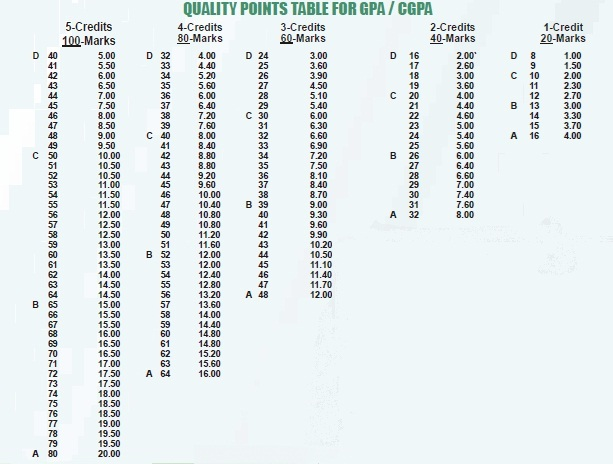GPA/CGPA will be calculated at the end of each semester in accordance to the following formula:

GPA/ CGPA = SUM OF QUALITY POINTS / SUM OF THE CREDIT HOURS

The quality points table given below will be used for calculating the GPA/CGPA: